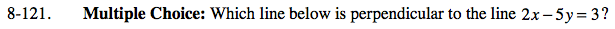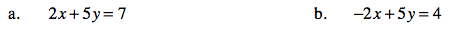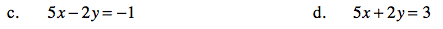### Home > CAAC > Chapter 8 > Lesson 8.3.3 > Problem8-121

8-121.Two lines are perpendicular if their slopes are negative reciprocals.

Put each equation, including the one in the problem, into slope intercept form.

Solve for y.

2x − 5y = 3

−5y = −2x = 3

$y=\frac{2}{5}x+\frac{3}{5}$

$m=\frac{2}{5}$

$\text{For a perpendicular line, }m=-\frac{5}{2}.$Solve for y.

Solve for y.Solve for y.

Solve for y.

Correct!